# Frequency Modulation (FM)

## Frequency Modulation (FM)

The frequency modulation (FM) is a type of angle modulation in which the instantaneous frequency fi(t) is varied in linear proportion with the instantaneous magnitude of the message signal x (t).

The mathematical expression for FM is as follows: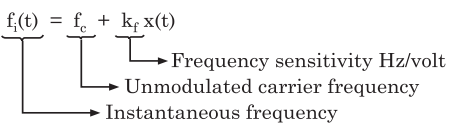In this expression fc denotes the frequency of unmodulated carrier and the constant krepresents the frequency sensitivity of the modulator. Its units are Hz/ volt, assuming the message signal to be a voltage signal.

Since the instantaneous frequency fi(t) of FM is changing continuously with time, we have to take the integration of x (t) over a duration of 0 to t.

Now we can write the expression of θ (t) for FM.

The expression for θ (t) of FM wave is given by: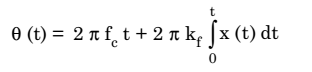### Mathematical Expression for FM

We can use θ (t) to write the expression for FM in time domain as under: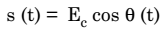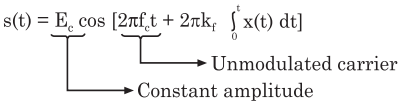### Relationship between FM and PM

The expressions for the FM and PM waves in the time domain are given by: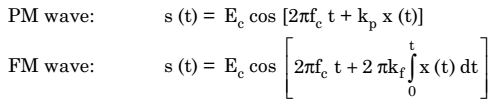### Generation of FM Using Phase Modulator

Comparing the above expressions, we can conclude that an FM wave is actually a PM wave having a modulating signal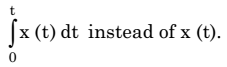This means that we can generate FM wave by applying the integrated version of x (t) to a phase modulator as shown in figure 1 below.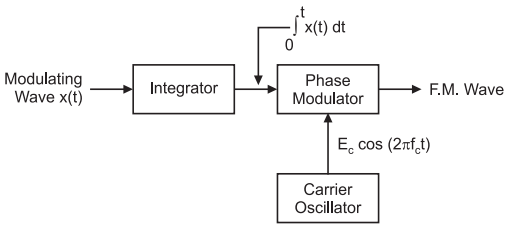Fig. 1: Generation of FM using Phase Modulator

Sharma, Dr Sanjay. Analog Communication Systems (Page 290). . Kindle Edition.